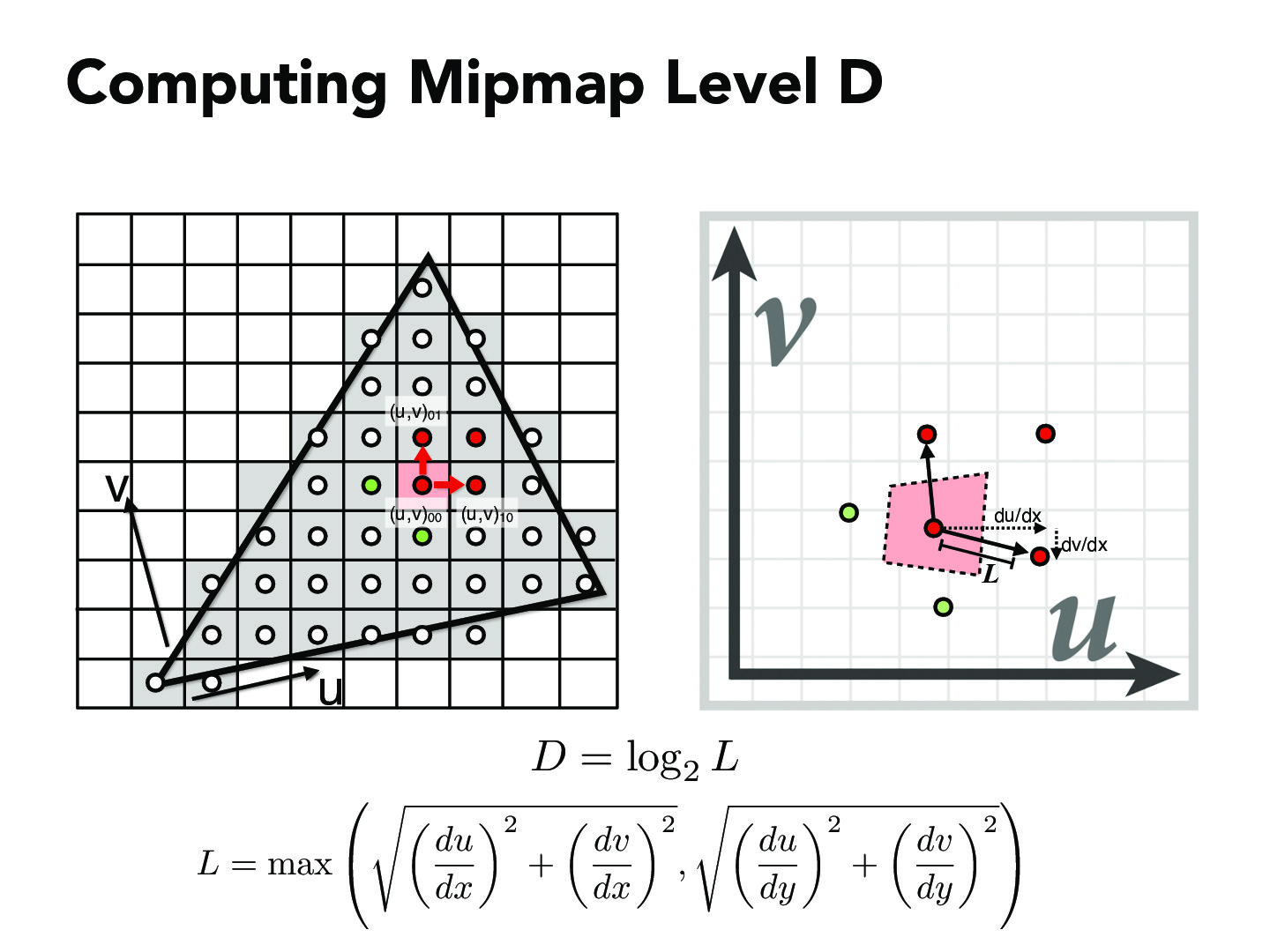Lecture 5: Texture Mapping (61)bufudash

Why do we use the du/dx, instead of u/x. Don't both of them describe the mapping from left to the right? Maybe it is because that x and y just indicates coordinates, and we are actually looking at delta x and delta y which is the length of the sides. Therefore, we should use derivatives.dangeng184

@bufudash, partial derivatives are useful because we're interested in the change of the u coord. given a change in the x coord. I think it also has to do with understanding how the (x,y) -> (u,v) transform affects the size of squares, which can be calculated by computing the determinant of the jacobian (which I think is what's approximately being done here, except they're dropping the sign). See (here)[https://en.wikipedia.org/wiki/Jacobian_matrix_and_determinant#Jacobian_determinant]

You must be enrolled in the course to comment